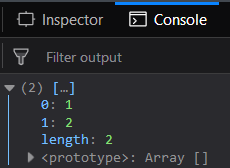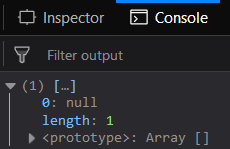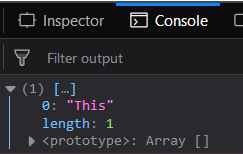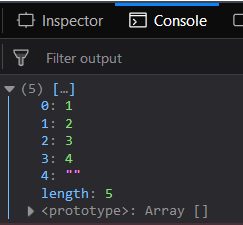Open In App
Related Articles

# Underscore.js _.intersection() Function

The Underscore.js is a JavaScript library that provides a lot of useful functions like the map, filter, invoke etc even without using any built-in objects.
The _.intersection() function is used to find the intersection of passed arrays, i.e. the elements which are common in all the n passed arrays in the _.intersection() function. This function find the elements which are present in all the arrays and then apply some operation on those elements then this function is used. It performs the intersection operation at the very basic level.
Syntax:

```_.intersection( *arrays )

```

Parameters: This function accepts single parameter arrays which contains set of array from which the common element need to be find.
Return value: It returns an array which contains the common elements of all the arrays.
Passing a list of numbers to _.intersection() function: The .intersection() function takes the element from the list one by one and then checks whether it is present in the list or not. If it is present in all the other arrays then only it will be included in the resultant array otherwise it is ignored.
Example:

 ```<``html``>``    ``<``head``>``        ``<``script` `src` `= ``"https://cdnjs.cloudflare.com/ajax/libs/underscore.js/1.9.1/underscore-min.js"` `>``        ````    ````    ``<``body``>``        ``<``script` `type``=``"text/javascript"``>``            ``console.log(_.intersection([1, 2, 3, 4, 5], ``                                       ``[1, 2, 3, 4, 6, 7], ``                                       ``[1, 2, 6, 8, 9])``            ``);``        ````    ````                    `

Output:Passing the false values to the _.intersection() function: If pass the false elements like the null, undefined along with the true elements like strings, numbers etc then the _.intersection() function will work in the same manner. The elements which are common inspite of being a false element will be in the resultant array.
Example:

 ```<``html``>``    ``<``head``>``        ``<``script` `src` `= ``"https://cdnjs.cloudflare.com/ajax/libs/underscore.js/1.9.1/underscore-min.js"` `>``        ````    ````    ``<``body``>``        ``<``script` `type``=``"text/javascript"``>``            ``console.log(_.intersection(["gfg", 52, " ", null], ``                                       ``[undefined, 4, null], ``                                       ``["", null],``                                       ``["gfg2", null])``            ``);``        ````    ````                    `

Output:Passing the words to the _.intersection() function: If pass the words like strings then the _.intersection() function will work in the same manner. The elements which are common inspite of being a string, empty string element will be in the resultant array. Like in the below example only the string “This” matches in all the arrays so it will be displayed.
Example:

 ```<``html``>``    ``<``head``>``        ``<``script` `src` `= ``"https://cdnjs.cloudflare.com/ajax/libs/underscore.js/1.9.1/underscore-min.js"` `>``        ````    ````    ``<``body``>``        ``<``script` `type``=``"text/javascript"``>``            ``console.log(_.intersection(["This", "geeks"], ``                              ``['for', "geeks2", "is", "amazing", "This"], ``                              ``["This", "is", "best", "platform"])``            ``);``        ````    ````                    `

Output:Passing the same array element to the _.intersection() function: Passing the arrays that have same elements then all the elements will be included in the resultant array. This is because all the elements are common to all the passed arrays.
Example:

 ```<``html``>``    ``<``head``>``        ``<``script` `src` `= ``"https://cdnjs.cloudflare.com/ajax/libs/underscore.js/1.9.1/underscore-min.js"` `>``        ````    ````    ``<``body``>``        ``<``script` `type``=``"text/javascript"``>``            ``console.log(_.intersection([1, 2, 3, 4, ""], ``                                       ``[1, 2, 3, 4, ""], ``                                       ``[1, 2, 3, 4, ""])``            ``);``        ````    ````                    `

Output:Note: These commands will not work in Google console or in Firefox as for these additional files need to be added which they didn’t have added. So, add the given links to your HTML file and then run them.

 `<``script` `type``=``"text/javascript"` `src` `= ``"https://cdnjs.cloudflare.com/ajax/libs/underscore.js/1.9.1/underscore-min.js"``> `` `